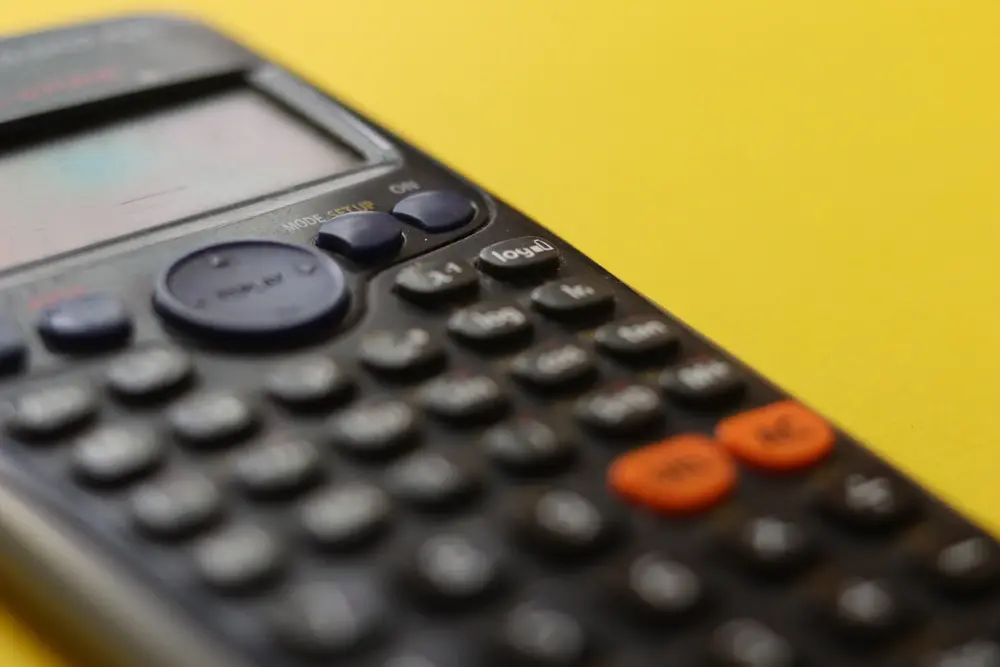# Iteration

This is a way of solving equations.

An iteration formula might look like the following:

Xn+1= 2 +  1
xn.

You are usually given a starting value, which is called x0. If x0 = 3, substitute 3 into the original equation where it says xn. This will give you x1. (This is because if n = 0, x1 = 2 + 1/x0 and x0 = 3).
x1 = 2 + 1/3 = 2.333 333 (by substituting in 3).
To find x2, substitute the value you found for x1.
x2 = 2 + 1/(2.333 333) = 2.428 571

Repeat this until you get an answer to a suitable degree of accuracy. This may be about the 5th value for an answer correct to 3s.f. In this example, x5 = 2.414...

Example
a) Show that x = 1 +   11
x - 3

is a rearrangement of the equation x² - 4x - 8 = 0.

b) Use the iterative formula Xn+1 = 1 +   11
xn - 3

together with a starting value of x1 = -2 to obtain a root of the equation x² - 4x - 8 = 0 accurate to one decimal place.

a) multiply everything by (x - 3):
x(x - 3) = 1(x - 3) + 11
so x² - 3x = x + 8
so x² - 4x - 8 = 0

b) x1 = -2
x2 = 1 +  11    (substitute -2 into the iteration formula)
-2 - 3
= -1.2

x3 = 1 +     11     (substitude -1.2 into the above formula)
-1.2 - 3

= -1.619
x4 = -1.381
x5 = -1.511
x6 = -1.439
x7 = -1.478
therefore, to one decimal place, x = 1.5

Numerical methods